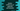# Difference between python append() and extend() methods of list## Difference between python append() and extend() methods of list:

append() and extend() are two predefined functions of python list. Both are used to add items to a list. But there is a difference between these two. In this post, we will learn how to use append() and extend() and the differences between them.

### append() :

append() method is used to add one item to the end of a list. This method is defined as below:

``list.append(e)``

It will append the element e to the list list. For example:

``````given_list = [1,2,3,4]

given_list.append(5)
print(given_list)

given_list.append('apple')
print(given_list)

given_list.append([5,6,7])
print(given_list)``````

In this example, we are appending items to the list given_list. We have three print statements in this program. It will print the below output:

``````[1, 2, 3, 4, 5]
[1, 2, 3, 4, 5, 'apple']
[1, 2, 3, 4, 5, 'apple', [5, 6, 7]]``````

The first one adds 5, the second one adds apple and the third one adds [5, 6, 7] to the original list given_list.

### extend() :

extend() method is used to add all elements of an iterable to the end of a list. For example :

``````given_list = [1,2,3,4]

given_list.extend([5,6,7])
print(given_list)``````

This will print out the below output:

``[1, 2, 3, 4, 5, 6, 7]``

Here, it adds all the elements of the second list to the origin list given_list at the end.